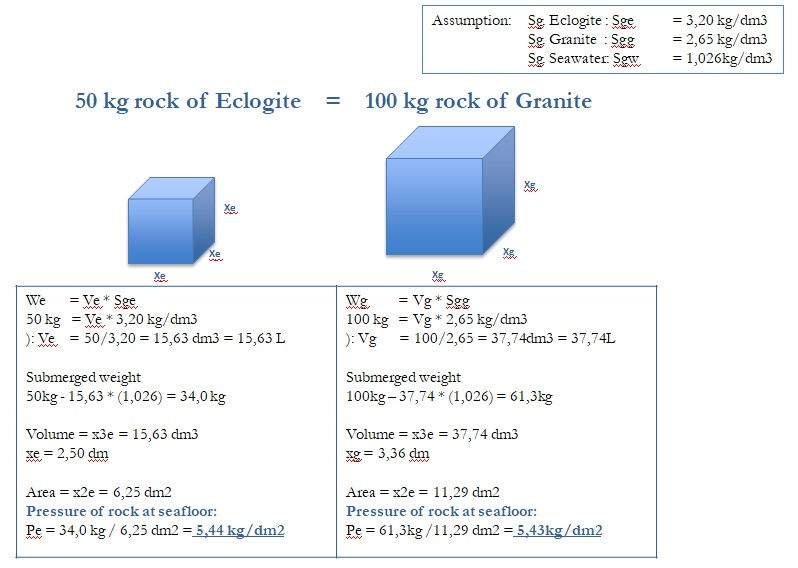top of page

## ECLOGITE VS GRANITE

The best way to fully understand the advantages of eclogite, it is crucial to compare it with another typical rock used in the North Sea.

The stone weight needed for a given rock density may be corrected according to the formula:

W=Wo       (Pso/Pw - 1) x Ps

(Ps /Pw - 1)  x Pso

W     =  Weight of actual rock

Wo   =  Weight of reference rock

Pw    =  Sea water density ~ 1.025 kg/dm

Ps     =  Actual rock density

Pso   =  Reference rock density = 2.68 kg/dm

and the stone weight is given by

W = Wo x k

The factor k below is shown as a function of the rock density.

To illustrate this formula, we compare 50 kg eclogite (of 3,2 t/m3) with 100 kg granite (of 2,65 t/m3).

Example:

W     =   Wo x k

W     =   Weight of Eclogite (density 3,20 kg/dm )

Wo   =   Weight of Granite (density 2,68 kg/dm )

k       =   Factor 0,50 (see graph)

W = 100 x 0,50 = 50 kg.

Conclusions:

A 100 kg Granite rock has the same submerged weight/dm2 as a 50 kg Eclogite rock.

A 100 kg Granite rock can be replaced with an Eclogite rock of 50 kg.bottom of page